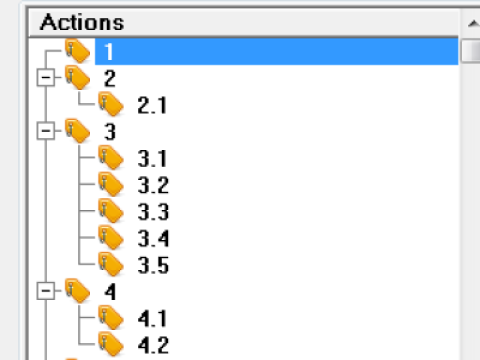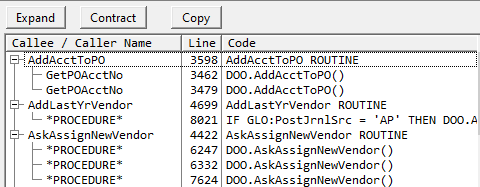# List Tree - How to Contract All Branches

How can I contract all nodes from picture. I want to see only root nodes (1, 2 3 4) ?
May be formatting with ?List{PROP:format}
Clarion 6.3This assumes your LevelField is 1 based.

``````loop curRow = 1 to records(TreeQueue)
get(TreeQueue, curRow)
if TreeQueue.LevelField > 1
TreeQueue.LevelField *= -1  ! make negative to close branch
put(TreeQueue)
end
end
``````

One Cosmetic flaw with that code is it makes ALL Branches Negative so ALL Contracted. The flaw is it will show a Contracted Box ( a Plus `[+]` ) on Branches that do not have lower levels. When the User clicks on the `[+]` nothing happens because there are no lower records. Like your first row “1” has nothing on level 2.

To prevent that only Contract branches that have lower levels, i.e. leave them as positive level numbers.

I coded that like below by LOOP in the Reverse “BY -1” to allow saving the previous lower level number.

``````TreeExpandContract (SomeQ, SomeQ:Level, True)   !Contract Tree
TreeExpandContract (SomeQ, SomeQ:Level, False)  !Expand Tree

TreeExpandContract PROCEDURE(QUEUE TreeQ, *LONG Level, BYTE Contract=0)
Prv LONG   !Keep Previous Record Level
CODE
LOOP X=RECORDS(TreeQ) TO 1 BY -1   !Reverse Loop Bottom to Top
GET(TreeQ,X)
Level=ABS(Level)
IF Level=1 THEN
IF Contract THEN
IF Prv=2 THEN Level=-1.
END
PUT(TreeQ)
END
Prv=Level
END
``````

Looking at the above code I think if it only works for a 2 Level Tree, which is what I had in the program I pulled it from. It also does not have a Root at Level 1. So you’ll need to adjust the code. Or maybe that does work for you as you only want to Contract Level 1.It works . Thank you both.